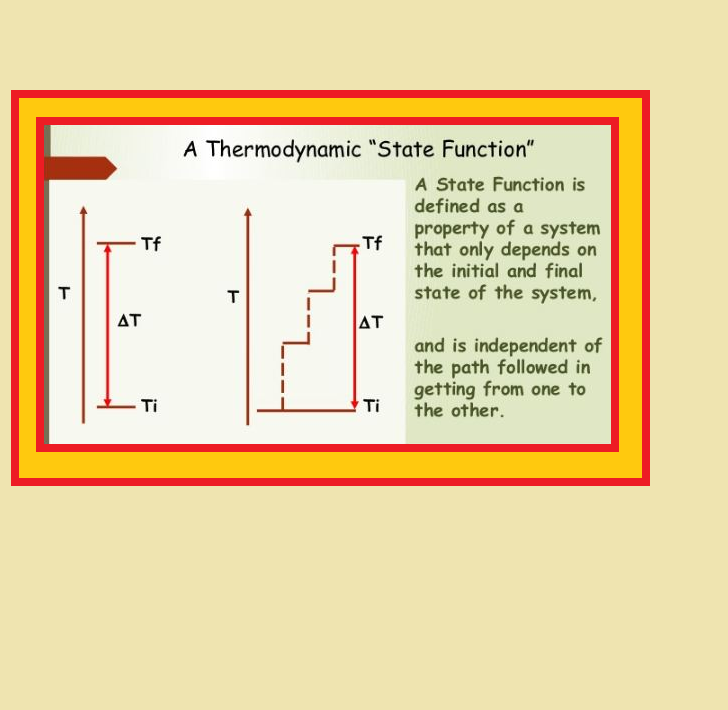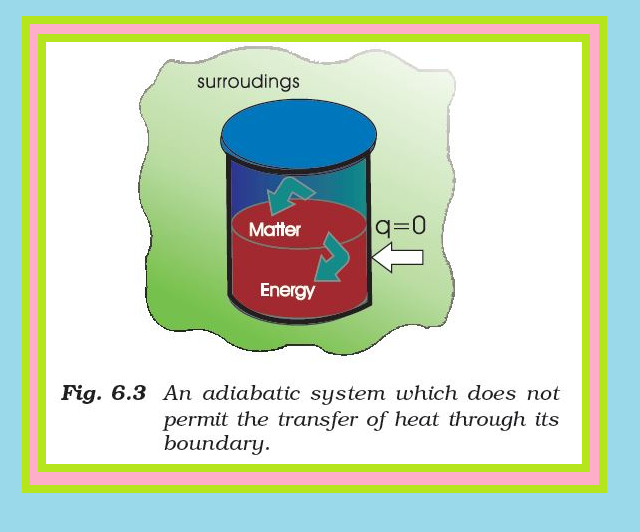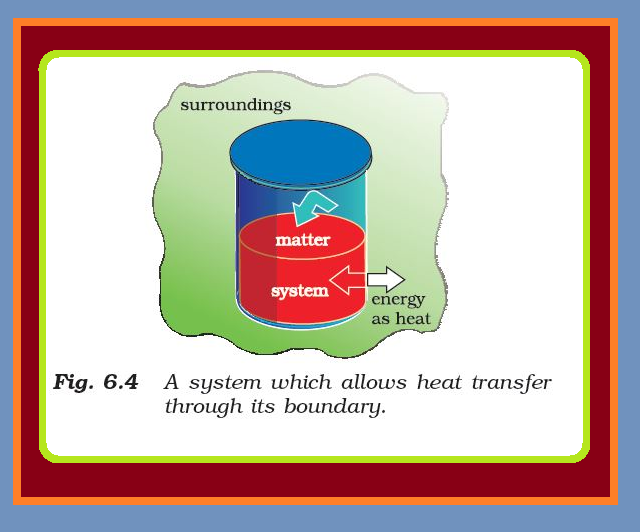Chemistry The State of the System and Internal Energy

### Topics Covered :

● The State of the System
● The Internal Energy as a State Function
● Work
● Heat
● The General Case

### The State of the System :=> The system must be described in order to make any useful calculations by specifying quantitatively each of the properties such as its pressure (p), volume (V), and temperature (T) as well as the composition of the system.

● We need to describe the system by specifying it before and after the change.

=> You would recall from your Physics course that the state of a system in mechanics is completely specified at a given instant of time, by the position and velocity of each mass point of the system.

=> In thermodynamics, a different and much simpler concept of the state of a system is introduced.

● It does not need detailed knowledge of motion of each particle because, we deal with average measurable properties of the system.

● We specify the state of the system by color{red}("state functions") or color{red}("state variables").

● The state of a thermodynamic system is described by its measurable or macroscopic (bulk) properties.

=> We can describe the state of a gas by quoting its pressure (p), volume (V), temperature (T), amount (n) etc.

● Variables like p, V, T are called color{red}("state variables") or color{red}("state functions") because their values depend only on the state of the system and not on how it is reached.

=> In order to completely define the state of a system it is not necessary to define all the properties of the system; as only a certain number of properties can be varied independently.

● This number depends on the nature of the system. Once these minimum number of macroscopic properties are fixed, others automatically have definite values.

color{red}("Note ") The state of the surroundings can never be completely specified; fortunately it is not necessary to do so.

### The Internal Energy as a State Function :

=> When we talk about our chemical system losing or gaining energy, we need to introduce a quantity which represents the total energy
of the system.

● It may be chemical, electrical, mechanical or any other type of energy you may think of, the sum of all these is the energy of the system.

● In thermodynamics, we call it the internal energy, U of the system, which may change, when

(i) heat passes into or out of the system,

(ii) work is done on or by the system,

(iii) matter enters or leaves the system.

### Work :=> Let us first examine a change in internal energy by doing work.

=> We take a system containing some quantity of water in a thermos flask or in an insulated beaker.

● This would not allow exchange of heat between the system and surroundings through its boundary and we call this type of system as color{green}("adiabatic"). The manner in which the state of such a system may be changed will be called text(adiabatic process).

color{green}("Adiabatic Process ") It is a process in which there is no transfer of heat between the system and surroundings.

● Here, the wall separating the system and the surroundings is called the color{red}("adiabatic wall") (Fig 6.3).

=> Let us bring the change in the internal energy of the system by doing some work on it.

● Let us call the initial state of the system as state A and its temperature as T_A.

● Let the internal energy of the system in state A be called U_A.

● We can change the state of the system in two different ways :

color{green}("One way" ) We do some mechanical work, say 1 kJ, by rotating a set of small paddles and thereby churning water. Let the new state be called B state and its temperature, as T_B.

● It is found that T_B > T_A and the change in temperature, ΔT = T_B–T_A.

● Let the internal energy of the system in state B be U_B and the change in internal energy, ΔU =U_B– U_A.

color{green}("Second way ") We now do an equal amount (i.e., 1 kJ) electrical work with the help of an immersion rod and note down the temperature change. We find that the change in temperature is same as in the earlier case, say, T_B – T_A.

=> In fact, the experiments in the above manner were done by J. P. Joule between 1840–50 and he was able to show that a given amount of work done on the system, no matter how it was done (irrespective of path) produced the same change of state, as measured by the change in the temperature of the system.

● So, it seems appropriate to define a quantity, the internal energy U, whose value is characteristic of the state of a system, whereby the adiabatic work, w_(ad) required to bring about a change of state is equal to the difference between the value of U in one state and that in another state, ΔU i.e.,

ΔU = U_2 −U_1 = w_(ad)

Therefore, internal energy, U, of the system is a state function.

=> The positive sign expresses that w_(ad) is positive when work is done on the system.

=> If the work is done by the system,w_(ad) will be negative.

=> Some of other familiar state functions are V, p, and T.

color{red}("Example" ) (i) If we bring a change in temperature of the system from 25°C to 35°C, the change in temperature is 35°C–25°C = +10°C, whether we go straight up to 35°C or we cool the system for a few degrees, then take the system to the final temperature. Thus, T is a state function and the change in temperature is independent of the route taken.

(ii) Volume of water in a pond, for example, is a state function, because change in volume of its water is independent of the route by which water is filled in the pond, either by rain or by tubewell or by both.

### Heat :=> We can also change the internal energy of a system by transfer of heat from the surroundings to the system or vice-versa without expenditure of work. This exchange of energy, which is a result of temperature difference is called color{red}("heat"), q.

=> Let us consider bringing about the same change in temperature (the same initial and final states as before in section 6.1.4 (a)) by transfer of heat through thermally conducting walls instead of adiabatic walls (Fig. 6.4).

=> We take water at temperature, T_A in a container having thermally conducting walls, say made up of copper and enclose it in a huge heat reservoir at temperature, T_B.

=> The heat absorbed by the system (water), q can be measured in terms of temperature difference, T_B – T_A.

● In this case, change in internal energy, ΔU= q, when no work is done at constant volume.

=> The q is positive, when heat is transferred from the surroundings to the system.

=> The q is negative when heat is transferred from system to the surroundings.

### The general case :

=> Let us consider the general case in which a change of state is brought about both by doing work and by transfer of heat.

● We write change in internal energy for this case as :

color{red}(ΔU = q + w) .............(6.1)

● For a given change in state, q and w can vary depending on how the change is carried out.

● However, color{red}(q + w = ΔU) will depend only on initial and final state. It will be independent of the way the change is carried out.

● If there is no transfer of energy as heat or as work (isolated system) i.e., if w = 0 and q = 0, then Δ U = 0.

● The equation 6.1 i.e., ΔU = q + w is mathematical statement of the text(first law of thermodynamics), which states that

color{green}("The energy of an isolated system is constant".)

● It is commonly stated as the law of conservation of energy i.e., energy can neither be created nor be destroyed.

color{red}("Note ") ● There is considerable difference between the character of the thermodynamic property energy and that of a mechanical property such as volume.

● We can specify an unambiguous (absolute) value for volume of a system in a particular state, but not the absolute value of the internal energy.

● However, we can measure only the changes in the internal energy, ΔU of the system.
Q 3027223181Express the change in internal energy of a system when

(i) No heat is absorbed by the system from the surroundings, but work (w) is done on the system. What type of wall does the system have ?

(ii) No work is done on the system, but q amount of heat is taken out from the system and given to the surroundings. What type of wall does the system have?

(iii) w amount of work is done by the system and q amount of heat is supplied to the system. What type of system would it be?Solution:

(i) Delta U = w_(ad), wall is adiabatic
(ii) Delta U = – q, thermally conducting walls
(iii) Delta U = q – w, closed system